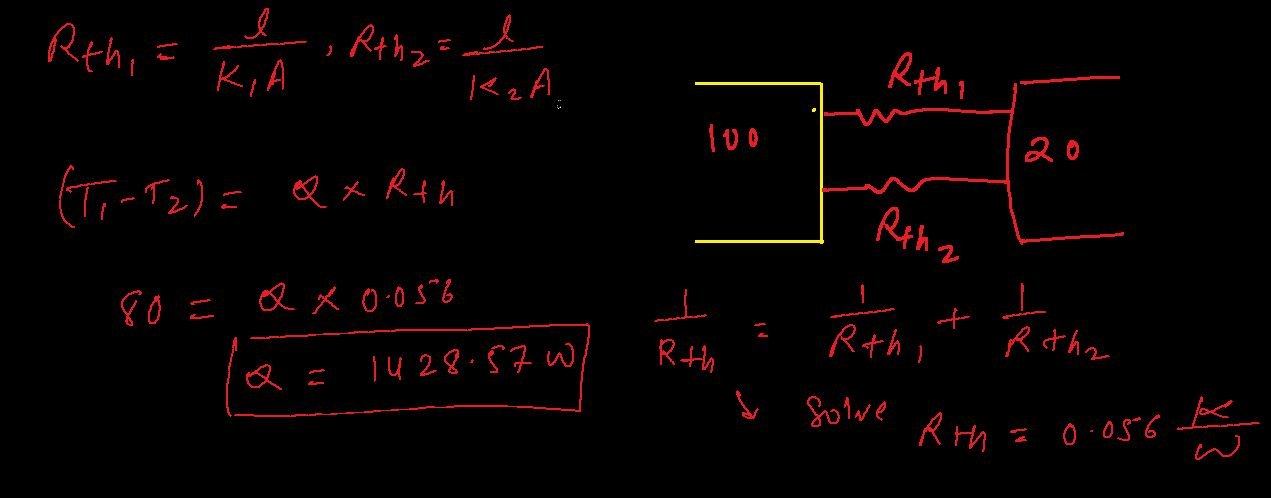×#### Thank you for registering.

One of our academic counsellors will contact you within 1 working day.

Click to Chat

1800-1023-196

+91-120-4616500

CART 0

• 0

MY CART (5)

Use Coupon: CART20 and get 20% off on all online Study Material

ITEM
DETAILS
MRP
DISCOUNT
FINAL PRICE
Total Price: Rs.

There are no items in this cart.
Continue Shopping
```
Two metal cubes with 3cm edges of copper and aluminum are arranged as shown if figure (k cu =385W/m-K,K Al =209W/m-K) then find the total thermal current from one reservoir to the other
Two metal cubes with 3cm edges of copper and aluminum are arranged as shown if figure (kcu=385W/m-K,KAl=209W/m-K) then find the total thermal current from one reservoir to the other

```
2 years ago

``````
2 years ago
```							Dear Student,Please find below the solution to your problem.Let the thermal resistance of Al cube be R1.Let the thermal resistance of Cu cube be R2.Applying the formula of thermal resistance,R = l/KAThermal resistance of Al will beR1 = l/KAR1 = 3 × (10^-2)/209 × (3 × 10^-2)^2R1 = 0.159 K/WCalculation of thermal resistance of CuR2 = l/KAR2 = 3 × (10^-2)/385 × (3 × 10^-2)^2R2 = 0.086 K/WFrom the figure we can clearly see that the given two resistances are connected in parallel way, so their equivalent resistance will be -R = (R1R2/R1 + R2)R = 0.159 × 0.086/(0.159 + 0.086)R = 0.055 K/Wthe total thermal current from one reservior to the other = Thermal Difference/Thermal Resistance= (100 – 20)/0.055= 1.45 × 10^3 WHence, the required answer is 1.45 × 10^3Thanks and Regards
```
5 months ago
Think You Can Provide A Better Answer ?

## Other Related Questions on Thermal Physics

View all Questions »### Course Features

• 101 Video Lectures
• Revision Notes
• Previous Year Papers
• Mind Map
• Study Planner
• NCERT Solutions
• Discussion Forum
• Test paper with Video Solution### Course Features

• 110 Video Lectures
• Revision Notes
• Test paper with Video Solution
• Mind Map
• Study Planner
• NCERT Solutions
• Discussion Forum
• Previous Year Exam Questions